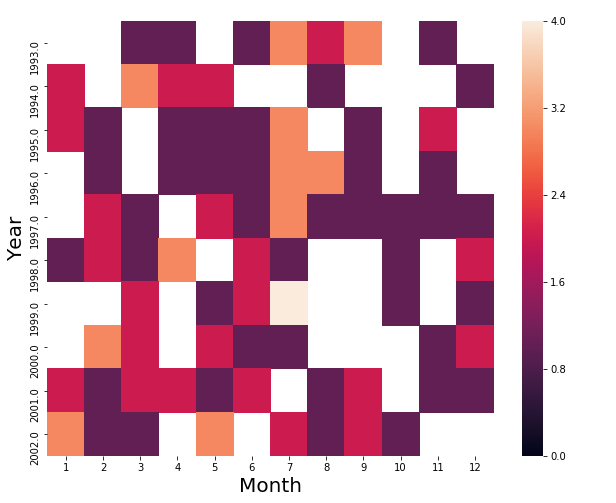﻿ Pandas Datetime: Create a heatmap for comparison of the top 10 years in which the UFO was sighted vs each Month - w3resource

# Pandas Datetime: Create a heatmap for comparison of the top 10 years in which the UFO was sighted vs each Month

## Pandas Datetime: Exercise-24 with Solution

Write a Pandas program to create a heatmap (rectangular data as a color-encoded matrix) for comparison of the top 10 years in which the UFO was sighted vs each Month.

Sample Solution:

Python Code:

``````import pandas as pd
import matplotlib.pyplot as plt
import seaborn as sns
#Source: https://bit.ly/1l9yjm9
df['Date_time'] = df['Date_time'].astype('datetime64[ns]')
def is_top_years(year):
if year in most_sightings_years.index:
return year
month_vs_year = df.pivot_table(columns=df['Date_time'].dt.month,index=df['Date_time'].dt.year.apply(is_top_years),aggfunc='count',values='city')
month_vs_year.columns = month_vs_year.columns.astype(int)
print("\nHeatmap for comparison of the top 10 years in which the UFO was sighted vs each month:")
plt.figure(figsize=(10,8))
ax = sns.heatmap(month_vs_year, vmin=0, vmax=4)
ax.set_xlabel('Month').set_size(20)
ax.set_ylabel('Year').set_size(20)
``````

Sample Output:

```Heatmap for comparison of the top 10 years in which the UFO was sighted vs each month:```

Python Code Editor:

Have another way to solve this solution? Contribute your code (and comments) through Disqus.

What is the difficulty level of this exercise?

﻿

Inviting useful, relevant, well-written and unique guest posts